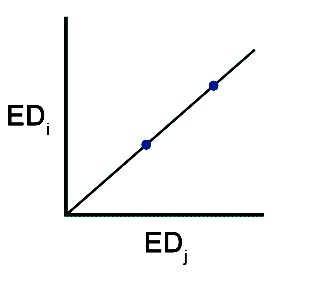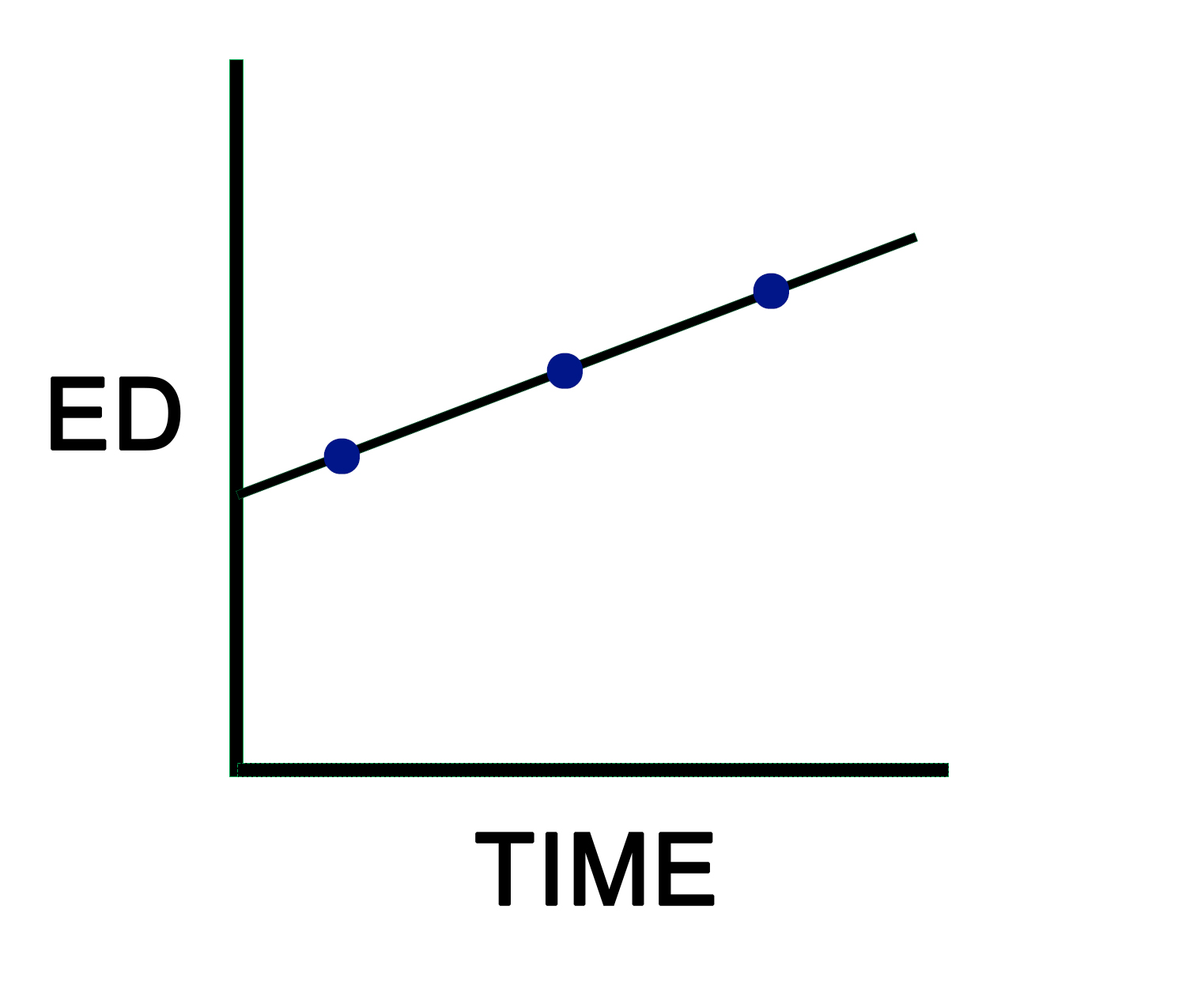## e-BOOK

Solving biology?  Impossible?  Not any more.  Since biology already knows the solutions, we’ll just copy them.  The primer explains how to do it.

## The Primer

Fortunately for us, biology has already solved itself as a mathematical problem.  The solution includes a theory structure (complexity), first principles, and rules.  This reduces the job of the primer to explaining how to copy biology’s solutions with equations.

For this to work, however, we’re required to play biology’s game according to biology’s rules.  This includes operating under a theory structure based on mathematics, which becomes our primary means of communication.  By speaking the same language, we can ask biology questions and expect answers in return.  An example will help.

Question: How does biology derive a change from first principles?

Answer: A biological change requires two first principles, which, in turn, it translates into a small army of rule-based equations.

1st Principle:    f(x) = mxThis first principle defines a linear equation passing through the origin, wherein each point on the line detects the same ratio of x to y (x:y).  In turn, biology uses teams of rules derived from this principle to produce structure-function recipes for its organelles.  Such recipes define the structural and functional complexities of organelles.

1st Principle:  f(x) = mx+bThe second principle also identifies a linear equation, but now the line no longer passes through the origin because the organelle recipe is undergoing complex changes.  This means that each point on the line has a different x to y ratio.  To an outsider, a biological change with all its complexity appears chaotic.  To biology, it’s a symphony being played flawlessly by a host of interacting rules and variables.

By applying these two first principles, biology can explain to us how it changes its phenotype in response to an experimental prod.  If, for purposes of illustration, we reduce our question about change to just one organelle with three of its marker enzymes, biology’s answer can be summarized with roughly 100 rule-based equations.

Notice the immediate benefit of our willingness to play by the rules.  By switching to biology’s theory structure, our answers experience a 25-fold increase in detail (100/4 = 25), along with many new insights.  Such a result is helpful because it means that we can solve the phenotype at a level of detail consistent with solving the genotype.  Recall that solving biology requires solving the complexity of both the phenotype and the genotype.

The primer offers the beginner a detailed look at the inner workings of biological complexity.  In reading the primer, two points quickly become apparent.  Biology’s approach to complexity is surprisingly easy to understand and duplicate because we’re no longer throwing away vital information.

Indeed, a rule-based approach to experimental biology makes our job easier, more enjoyable, and highly productive.

## What’s inside

^

COMPLEXITY

^

EQUATIONS

^

#### Chapter 3

VISUALIZING COMPLEXITY

^

REPRODUCIBILITY

^

DATA

^

DATABASES

^

CALCULATIONS

^

#### Chapter 8

HOW TO SOLVE BIOLOGY

^

#### Chapter 9

COMPLEXITY THEORY

##### COMPLEXITY

Solving biology is an exercise in solving complexities by creating complexities. The process consists of using published data to create complexities parallel to the ones used by biology to solve a given problem. In effect, we recruit biology to solve our problems for us, being confident that it has already worked out the best solutions. We can safely assume that biology is qualified to do the heavy lifting because it knows what rules to apply and how to summon the necessary resources. It understands full well that survival depends on its ability to adapt quickly and effectively.

Chapter 1, which introduces the first six levels of complexity, tells the story simply and succinctly. Although touching on the central themes and principal findings, it keeps details to a minimum. Getting the big picture at the outset will make it easier for us to tackle the specifics in the ensuing chapters.

### Pages

The primer explains how to copy biology as it exists in nature . . .

“Study nature, not books.”
Louis Agassiz

“. . . what I tell you three times is true.”
Lewis Carroll

## First book in the series

#### Preface

“Here is the problem. We have a science – called biology – that lacks a mathematical foundation and can produce data so corrupted by bias and biological variation that the original information often becomes unrecognizable. To make matters worse, we assume that we can study biology by reducing its complexity to a simplicity, characterize its parts in isolation, and then use the resulting information to explain biology as it normally exists. We dig the hole even deeper by assuming that our methods allow us to detect biological changes, when often the best they can do is detect significant differences between heavily biased data sets. Consequently, the data we publish all too often stand little chance of representing biology, as it is.”